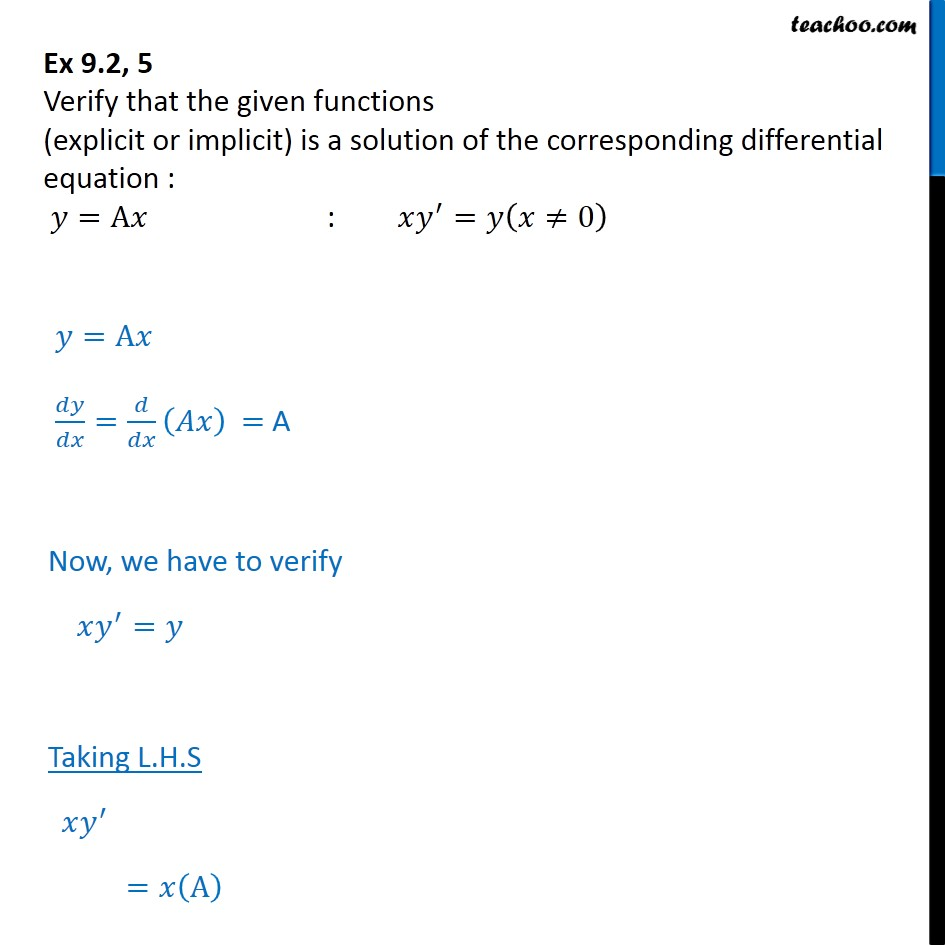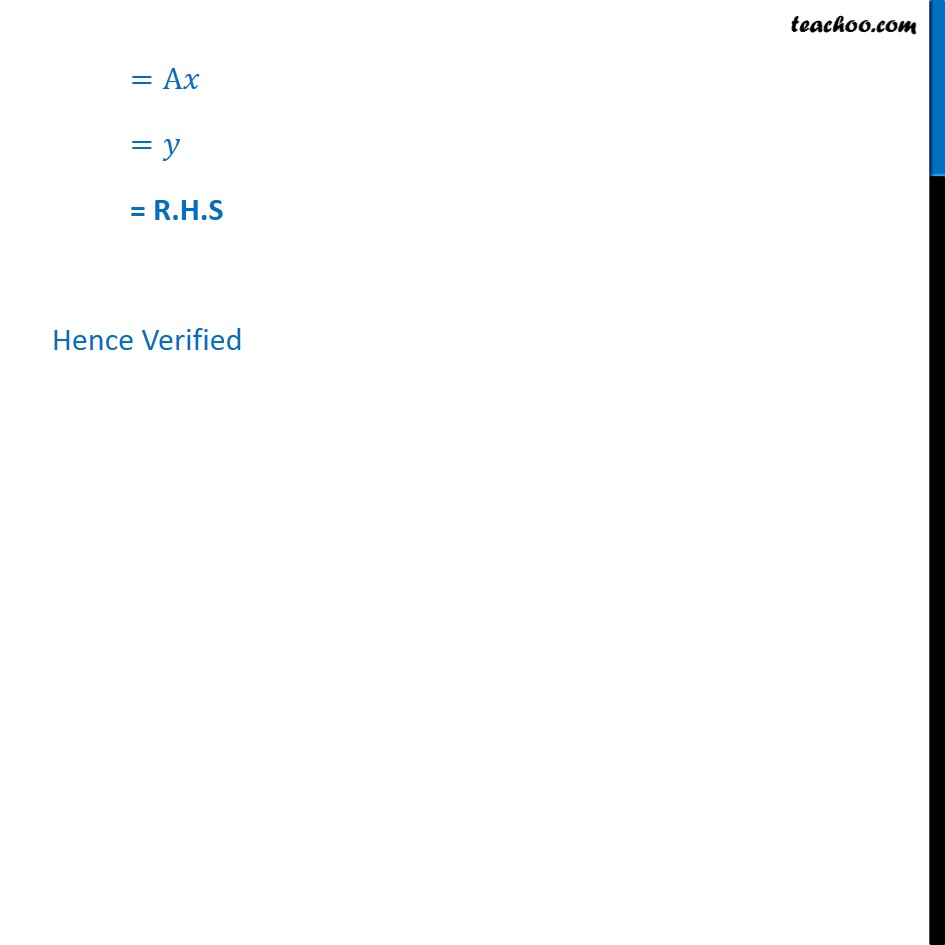Ex 9.2

Chapter 9 Class 12 Differential Equations
Serial order wiseLearn in your speed, with individual attention - Teachoo Maths 1-on-1 Class

### Transcript

Ex 9.2, 5 Verify that the given functions (explicit or implicit) is a solution of the corresponding differential equation : =A : = 0 =A = = A Now, we have to verify = Taking L.H.S = A =A = = R.H.S Hence Verified